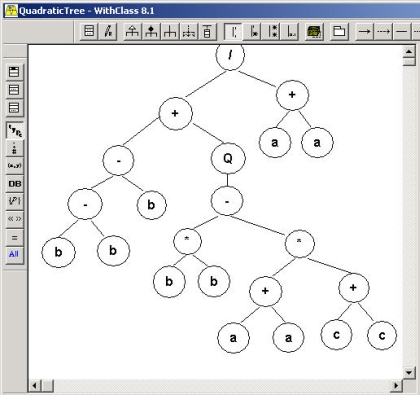CodeKicks.com Focus on Microsoft Technologies - Tutorials, Articles, Code Samples.

## Monday, October 12, 2009

### Gene Expression Programming (GEP) in C# and .NET

In the past we explored ways of using Genetic Algorithms (GA) to solve problems with a string of numbers that represent numbers. For example, we used genetic algorithms in our MasterMind game as a computer player for solving the hidden color combination where each color was represented by an integer. Gene Expression programming (GEP) is also a subset of Genetic Algorithms, except it uses genomes whose strings of numbers represent symbols.  The string of symbols can further represent equations, grammars, or logical mappings.  The genome can be mapped to a binary tree that you can walk along the nodes to evaluate the equation.  This is an extraordinarily powerful technique because now you are not mapping a GA to hard values, but to general symbols.Figure 1 - Quadratic Equation represented in a symbolic tree expression

Let's give an example of what we mean.  Lets let the value 0 represent the variable a,  1 represent multiplication, and 2 represent +.  So the string of numbers below:Continue